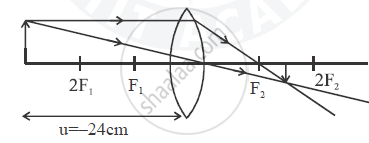# An Object is Placed at a Distance 24 Cm in Front of a Convex Lens of Focal Length 8 Cm.(I) What is the Nature of the Image So Formed ? (Ii) Calculate the Distance of the Image from the Lens. - Physics

Short Note

An object is placed at a distance 24 cm in front of a convex lens of focal length 8 cm.

(i) What is the nature of the image so formed ?
(ii) Calculate the distance of the image from the lens.
(iii) Calculate the magnification of the image.

#### Solution(i) As for convex lens, if |u| > | f | then real and inverted image is formed and as |u| > 2| f | then it is diminished .

(ii)1/v - 1/u = 1 /f

1/v = 1/u + 1 /f

1/v =(f+u)/(uf)

⇒ v =(uf)/(f+u)= ((-24)(8))/((8)+(-24)) = (-24xx8)/(-162) = 12 cm

(iii) m = v/u = 12/-24 = -0.5

-ve sign signifies inverted image.

Concept: Concave Lens
Is there an error in this question or solution?